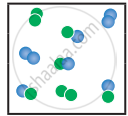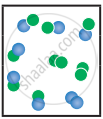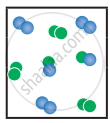Tamil Nadu Board of Secondary EducationHSC Science Class 11th

# Tamil Nadu Board Samacheer Kalvi solutions for Class 11th Chemistry Volume 1 and 2 Answers Guide chapter 8 - Physical and Chemical Equilibrium [Latest edition]

#### Chapters## Chapter 8: Physical and Chemical Equilibrium

Evaluation
Evaluation [Pages 22 - 28]

### Tamil Nadu Board Samacheer Kalvi solutions for Class 11th Chemistry Volume 1 and 2 Answers Guide Chapter 8 Physical and Chemical Equilibrium Evaluation [Pages 22 - 28]

Evaluation | Q I. 1. | Page 22

If Kb and Kf for a reversible reaction are 0.8 × 10-5 and 1.6 × 10-4 respectively, the value of the equilibrium constant is,

• 20

• 0.2 × 10-1

• 0.05

• None of these

Evaluation | Q I. 2. | Page 22

At a given temperature and pressure, the equilibrium constant values for the equilibria

$\ce{3A2 + B2 + 2Cr <=>[K1] 2A3BC}$ and

$\ce{A3BC <=> 3/2 A2 + 1/2 B2 + C}$

The relation between K1 and K2 is

• "K"_1 = 1/sqrt"K"_2

• "K"_2 = "K"_1^(-1//2)

• "K"_1^2 = 2"K"_2

• "K"_1/2 = "K"_2

Evaluation | Q I. 3. | Page 22

The equilibrium constant for a reaction at room temperature is K1 and that at 700 K is K2 If K1 > K2, then

• The forward reaction is exothermic

• The forward reaction is endothermic

• The reaction does not attain equilibrium

• The reverse reaction is exothermic

Evaluation | Q I. 4. | Page 23

The formation of ammonia from N2(g) and H2(g) is a reversible reaction

$\ce{N2(g) + 3H2(g) <=> 2NH3(g) + Heat}$

What is the effect of increase of temperature on this equilibrium reaction.

• equilibrium is unaltered

• formation of ammonia is favoured

• equilibrium is shifted to the left

• reaction rate does not change

Evaluation | Q I. 5. | Page 23

Solubility of carbon dioxide gas in cold water can be increased by ______.

• increase in pressure

• decrease in pressure

• increase in volume

• none of these

Evaluation | Q I. 6. | Page 23

Which one of the following is incorrect statement ?

• for a system at equilibrium, Q is always less than the equilibrium constant

• equilibrium can be attained from either side of the reaction

• presence of catalyst affects both the forward reaction and reverse reaction to the same extent

• Equilibrium constant varied with temperature

Evaluation | Q I. 7. | Page 23

K1 and K2 are the equilibrium constants for the reactions respectively.

$\ce{N2(g) + O2(g) <=>[K1] 2NO(g)}$

$\ce{NO(g) + O2(g) <=>[K2] 2NO2(g)}$

What is the equilibrium constant for the reaction $\ce{NO2(g) <=> 1/2 N2(g) + O2(g)}$

• 1/(sqrt("K"_1 "K"_2))

• (K1 = K2)1/2

• 1/(2"K"_1"K"_2)

• (1/("K"_1"K"_2))^(3//2)

Evaluation | Q I. 8. | Page 23

In the equilibrium,

$\ce{2A(g) <=> 2B(g) + C2(g)}$

the equilibrium concentrations of A, B and C2 at 400 K are 1 × 10–4 M, 2.0 × 10–3 M, 1.5 × 10–4 M respectively. The value of KC for the equilibrium at 400 K is

• 0.06

• 0.09

• 0.62

• 3 × 10-2

Evaluation | Q I. 9. | Page 23

An equilibrium constant of 3.2 × 10-6 for a reaction means, the equilibrium is

• largely towards forward direction

• largely towards reverse direction

• never established

• none of these

Evaluation | Q I. 10. | Page 23

"K"_"C"/"K"_"P" for the reaction,

$\ce{N2(g) + 3H2(g) <=> 2NH3(g)}$ is

• 1/"RT"

• sqrt"RT"

• RT

• (RT)2

Evaluation | Q I. 11. | Page 23

For the reaction $\ce{AB(g) <=> A(g) + B(g)}$, at equilibrium, AB is 20 % dissociated at a total pressure of P, the equilibrium constant Kp is related to the total pressure by the expression

• P = 24 Kp

• P = 8 Kp

• 24 P = Kp

• none of these

Evaluation | Q I. 12. | Page 24

In which of the following equilibrium, Kp and Kc are not equal?

• $\ce{2NO(g) <=> N2(g) + O2(g)}$

• $\ce{SO2(g) + NO2 <=> SP3(g) + NO(g)}$

• $\ce{H2(g) + I2(g) <=> 2HI(g)}$

• $\ce{PCl5(g) <=> PCl3(g) + Cl2 (g)}$

Evaluation | Q I. 13. | Page 24

If x is the fraction of PCl5 dissociated at equilibrium in the reaction

$\ce{PCl5 <=> PCl3 + Cl2}$

then starting with 0.5 mole of PCl5, the total number of moles of reactants and products at equilibrium is

• 0.5 – x

• x + 0.5

• 2x + 0.5

• x + 1

Evaluation | Q I. 14. | Page 24

The values of Kp1 and Kp2; for the reactions,

X ⇌ Y + Z,

A ⇌ 2B are in the ratio 9 : 1 if degree of dissociation of X and A be equal then total pressure at equilibrium P1, and P2 are in the ratio

• 36 : 1

• 1 : 1

• 3 : 1

• 1 : 9

Evaluation | Q I. 15. | Page 24

In the reaction

$\ce{Fe(OH)3(S) <=> Fe^{(3+)} (aq) + 3OH- (aq)}$

if the concentration of OH ions is decreased by 1/4 times, then the equilibrium concentration of Fe3+ will

• not changed

• also decreased by 1/4 times

• increase by 4 times

• increase by 64 times

Evaluation | Q I. 16. | Page 24

Consider the reaction where Kp = 0.5 at a particular temperature

$\ce{PCl5(g) <=> PCl3 (g) + Cl2 (g)}$

if the three gases are mixed in a container so that the partial pressure of each gas is initially 1 atm, then which one of the following is true.

• more PCl3 will be produced

• more Cl2 will be produced

• more PCl5 will be produced

• None of these

Evaluation | Q I. 17. | Page 24

Equimolar concentrations of H2 and I2 are heated to equilibrium in a 1 liter flask. What percentage of the initial concentration of H2 has reacted at equilibrium if the rate constant for both forward and reverse reactions are equal

• 33%

• 66%

• (33)2%

• 16.5%

Evaluation | Q I. 18. | Page 24

In a chemical equilibrium, the rate constant for the forward reaction is 2.5 × 10-2, and the equilibrium constant is 50. The rate constant for the reverse reaction is,

• 11.5

• 50

• 2 × 102

• 2 × 10-3

Evaluation | Q 19. | Page 24

Which of the following is not a general characteristic of equilibrium involving physical process

• Equilibrium is possible only in a closed system at a given temperature

• The opposing processes occur at the same rate and there is a dynamic but stable condition

• All the physical processes stop at equilibrium

• All measurable properties of the system remains constant

Evaluation | Q 20. | Page 25

For the formation of Two moles of SO3(g) from SO2 and O2, the equilibrium constant is K1. The equilibrium constant for the dissociation of one mole of SO3 into SO2 and O2 is

• 1/"K"_1

• "K"_1^2

• (1/"K"_1)^(1/2)

• "K"_1/2

Evaluation | Q 21. | Page 25

Match the equilibria with the corresponding conditions:

1. Liquid ⇌ Vapour
2. Solid ⇌ Liquid
3. Solid ⇌ Vapour
4. Solute(s) ⇌ Solute (Solution)
1. Melting point
2. Saturated solution
3. Boiling point
4. Sublimation point
5. Unsaturated solution
• (i) - 1, (ii) - 2, (iii) - 3, (iv) - 4

• (i) - 3, (ii) - 1, (iii) - 4, (iv) - 2

• (i) - 2, (ii) - 1, (iii) - 3, (iv) - 4

• (i) - 3, (ii) - 2, (iii) - 4, (iv) - 5

Evaluation | Q 22. | Page 25

Consider the following reversible reaction at equilibrium,

$\ce{A + B <=> C}$,

If the concentration of the reactants A and B are doubled, then the equilibrium constant will

• be doubled

• become one fourth

• be halved

• remain the same

Evaluation | Q 23. | Page 25

$\ce{[CO(H2O)6]^2+ (aq) (pink) + 4Cl- (aq) <=> [CoCl4]^2- (aq) (blue) + 6 H2O (l)}$

In the above reaction at equilibrium, the reaction mixture is blue in colour at room temperature. On cooling this mixture, it becomes pink in color. On the basis of this information, which one of the following is true?

• ∆H > 0 for the forward reaction

• ∆H = 0 for the reverse reaction

• ∆H < 0 for the forward reaction

• Sign of the ∆H cannot be predicted based on this information

Evaluation | Q 24. | Page 25

The equilibrium constants of the following reactions are:

$\ce{N2 + 3H2 <=> 2NH3}$; K1

$\ce{N2 + O2 <=> 2NO}$; K2

$\ce{H2 + 1/2O2 <=> H2O}$; K3

The equilibrium constant (K) for the reaction;

$\ce{2NH3 + 5/2 O2 <=> 2NO + 3H2O}$, will be

• "K"_2^3  "K"_3/"K"_1

• "K"_1  "K"_3^3/"K"_2

• "K"_2  "K"_3^3/"K"_1

• "K"_2  "K"_3/"K"_1

Evaluation | Q 25. | Page 26

A 20 litre container at 400 K contains CO2 (g) at pressure 0.4 atm and an excess (neglect the volume of solid SrO). The volume of the container is now decreased by moving the movable piston fitted in the container. The maximum volume of the container, when the pressure of CO2 attains its maximum value will be:

Given that: $\ce{SeCO3(S)≅ SrO + CO2 (g)}$ [Kp = 1.6 atm]

• 2 litre

• 5 litre

• 10 litre

• 4 litre

#### Write brief answer to the following questions.

Evaluation | Q II. 1. | Page 26

If there is no change in concentration, why is the equilibrium state considered dynamic?

Evaluation | Q II. 2 | Page 26

For a given reaction at a particular temperature, the equilibrium constant has a constant value. Is the value of Q also constant? Explain.

Evaluation | Q II. 3. | Page 26

What is the relation between Kp and Kc? Given one example for which Kp is equal to Kc.

Evaluation | Q II. 4. | Page 26

For a gaseous homogeneous reaction at equilibrium, a number of moles of products is greater than the number of moles of reactants. Is KC is larger or smaller than Kp?

Evaluation | Q II. 5. | Page 26

When the numerical value of the reaction quotient (Q) is greater than the equilibrium constant, in which direction does the reaction proceed to reach equilibrium?

Evaluation | Q II. 6. | Page 26

For the reaction, $\ce{A2(g) + B2(g) <=> 2AB(g); \Delta H}$ is -ve.

the following molecular scenes represent differenr reaction mixture. (A-green, B-blue)

 Closed ←System At equilibrium (x) (y)
1. Calculate the equilibrium constant Kp and (Kc).
2. For the reaction mixture represented by scene (x), (y) the reaction proceed in which directions?
3. What is the effect of an increase in pressure for the mixture at equilibrium?
Evaluation | Q II. 7. | Page 26

State Le – Chateller principle.

Evaluation | Q II. 8. a) | Page 26

Consider the following reaction,

$\ce{H2(g) + l2(g) <=> 2HI(g)}$

In the above reactions find out whether you have to increase (or) decrease the volume to increase the yield of the product.

Evaluation | Q II. 8. b) | Page 26

Consider the following reaction,

$\ce{CaCO3(s) <=> CaO(s) + CO2(g)}$

In the above reactions find out whether you have to increase (or) decrease the volume to increase the yield of the product.

Evaluation | Q II. 8. c) | Page 26

Consider the following reaction,

$\ce{S(s) + F2(g) <=> SF6(g)}$

In the above reactions find out whether you have to increase (or) decrease the volume to increase the yield of the product.

Evaluation | Q II. 9. | Page 26

State law of mass action.

Evaluation | Q II. 10. | Page 26

Explain how will you predict the direction of an equilibrium reaction.

Evaluation | Q II. 11. | Page 27

Derive a general expression for the equilibrium constant Kp and Kc for the reaction, $\ce{3H2(g) + N2(g) <=> 2NH3(g)}$.

Evaluation | Q II. 12. | Page 27

Write the balanced chemical equation for an equilibrium reaction for which the equilibrium constant is given by expression.

"K"_"C" = (["NH"_3]^4["O"_2]^5)/(["NO"]^4["H"_2"O"]^6)

Evaluation | Q II. 13. | Page 27

What is the effect of added Inert gas on the reaction at equilibrium?

Evaluation | Q II. 14. | Page 27

Derive the relation between Kp and Kc.

Evaluation | Q II. 15. | Page 27

One mole of PCl5 is heated in one litre closed container. If 0.6 mole of chlorine is found at equilibrium, Calculate the value of equilibrium constant.

Evaluation | Q II. 16. | Page 27

For the reaction

$\ce{SrCO3(s) <=> SrO(s) + CO2(g)}$

the value of equilibrium constant Kp = 2.2 × 10-4 at 1002 K. Calculate Kc for the reaction.

Evaluation | Q II. 17. | Page 27

To study the decomposition of hydrogen iodide, a student fills an evacuated 3 litre flask with 0.3 mol of HI gas and allows the reaction to proceed at 500°C. At equilibrium he found the concentration of HI which is equal to 0.05 M. Calculate Kc and Kp.

Evaluation | Q II. 18 | Page 27

1 mol of CH4, 1 mole of CS2 and 2 mol of H2S are 2 mol of H2 are mixed in a 500 ml flask. The equilibrium constant for the reaction Kc = 4 x 10-2 mol2 lit-2. In which direction will the reaction proceed to reach equilibrium?

Evaluation | Q II. 19. i) | Page 27

At particular temperature Kc = 4 × 10-2 for the reaction, $\ce{H2S (g) <=> H2(g) +1/2 S2(g)}$. Calculate the Kc for the following reaction.

$\ce{2H2S (g) <=> 2H2 (g) + S2 (g)}$

Evaluation | Q II. 19. ii) | Page 27

At particular temperature Kc = 4 × 10-2 for the reaction, $\ce{H2S (g) <=> H2(g) +1/2 S2(g)}$. Calculate the Kc for the following reaction.

$\ce{3H2S (g) <=> 3H2 (g) + 3/2 S2 (g)}$

Evaluation | Q II. 20. | Page 27

28 g of Nitrogen and 6 g of hydrogen were mixed In a 1 litre closed container. At equIlibrium 17 g NH3 was produced. Calculate the weight of nitrogen, hydrogen at equilibrium.

Evaluation | Q II. 21 | Page 27

The equilibrium for the dissociation of XY2 is given as,

$\ce{2 XY2 (g) <=> 2 XY (g) + Y2 (g)}$

if the degree of dissociation x is so small compared to one. Show that 2 Kp = PX3 where P is the total pressure and Kp is the dissociation equilibrium constant of XY2.

Evaluation | Q II. 22 | Page 27

A sealed container was filled with 0.3 mol H2(g), 0.4 mol I2(g) and 0.2 mol HI(g) at 800 K and total pressure 1.00 bar. Calculate the amounts of the components in the mixture at equilibrium given that K = 870 for the reaction, $\ce{A2 (g) + B2 (g) <=> 2 AB (g)}$.

Evaluation | Q II. 23. | Page 27

Deduce the Vant Hoff equation.

Evaluation | Q II. 24 | Page 27

The equilibrium constant Kp for the reaction $\ce{N2 (g) + 3H2 (g) <=> 2NH3 (g)}$ is 8.19 × 102 at 298 K and 4.6 × 10-1 at 498 K. Calculate ∆H° for the reaction.

Evaluation | Q II. 25 | Page 28

The partial pressure of carbon dioxide in the reaction

$\ce{CaCO3(s) <=> CaO(s) + CO2(g)}$ is 1.017 × 10-3 atm at 500°C. Calculate Kp at 600°C for the reaction. H for the reaction is 181 KJ mol-1 and does not change in the given range of temperature.

## Chapter 8: Physical and Chemical Equilibrium

Evaluation## Tamil Nadu Board Samacheer Kalvi solutions for Class 11th Chemistry Volume 1 and 2 Answers Guide chapter 8 - Physical and Chemical Equilibrium

Tamil Nadu Board Samacheer Kalvi solutions for Class 11th Chemistry Volume 1 and 2 Answers Guide chapter 8 (Physical and Chemical Equilibrium) include all questions with solution and detail explanation. This will clear students doubts about any question and improve application skills while preparing for board exams. The detailed, step-by-step solutions will help you understand the concepts better and clear your confusions, if any. Shaalaa.com has the Tamil Nadu Board of Secondary Education Class 11th Chemistry Volume 1 and 2 Answers Guide solutions in a manner that help students grasp basic concepts better and faster.

Further, we at Shaalaa.com provide such solutions so that students can prepare for written exams. Tamil Nadu Board Samacheer Kalvi textbook solutions can be a core help for self-study and acts as a perfect self-help guidance for students.

Concepts covered in Class 11th Chemistry Volume 1 and 2 Answers Guide chapter 8 Physical and Chemical Equilibrium are Physical and Chemical Equilibrium, Introduction of Chemical Equilibrium, Homogeneous and Heterogeneous Equilibria, Equilibrium Constants, Applications of Equilibrium Constants, Le-Chatelier's Principle, Van't Hoff Equation.

Using Tamil Nadu Board Samacheer Kalvi Class 11th solutions Physical and Chemical Equilibrium exercise by students are an easy way to prepare for the exams, as they involve solutions arranged chapter-wise also page wise. The questions involved in Tamil Nadu Board Samacheer Kalvi Solutions are important questions that can be asked in the final exam. Maximum students of Tamil Nadu Board of Secondary Education Class 11th prefer Tamil Nadu Board Samacheer Kalvi Textbook Solutions to score more in exam.

Get the free view of chapter 8 Physical and Chemical Equilibrium Class 11th extra questions for Class 11th Chemistry Volume 1 and 2 Answers Guide and can use Shaalaa.com to keep it handy for your exam preparation